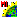# vnode.sml

###Download

#### Syntax Highlighing:

```comments, key words, predefined symbols, class members & methods, functions & classes
```
```# vnode.sml
clear();
vector V;
array numeric xPoints, yPoints, nodeList;
numeric numPoints;
numeric x, y;
numeric searchDistance;
numeric node1, numNodes;
GetOutputVector(V, "VectorToolkit");
#initialize some points for our polygon box
xPoints = 0;
yPoints = 0;
xPoints = 70;
yPoints = 70;
xPoints = 90;
yPoints = 100;
xPoints = 130;
yPoints = 120;
# create a line
numPoints = 4;
VectorAddLine(V, numPoints, xPoints, yPoints);
# create a second line
numPoints = 4;
numeric i;
for i = 1 to 5 {
xPoints[i] = xPoints[i] + 200;
}
VectorAddLine(V, numPoints, xPoints, yPoints);
# now add a some nodes to the first line
x = 10;
y = 10;
searchDistance = 10;
for i = 1 to 4 {
VectorAddNode(V, x, y, searchDistance);
print("node added at x, y: ", x, y);
x = x + 10;
y = y + 10;
}
# now add a some nodes to the second line
x = 210;
y = 10;
searchDistance = 10;
for i = 1 to 4 {
VectorAddNode(V, x, y, searchDistance);
print("node added at x, y: ", x, y);
x = x + 10;
y = y + 10;
}

# now delete a single node from the first line
node1 = FindClosestNode(V, 10, 10);
VectorDeleteNode(V, node1);
print("node", node1, "at x, y", 10, 10, "will be deleted");
# now delete multiple nodes from the first line
x = 20;
y = 20;
searchDistance = 10;
for i = 1 to 3 {
nodeList[i] = FindClosestNode(V, x, y);
print("node", nodeList[i], "at x, y", x, y, "will be deleted");
x = x + 10;
y = y + 10;
}
numNodes = 3;
VectorDeleteNodes(V, nodeList, numNodes);```